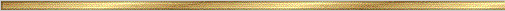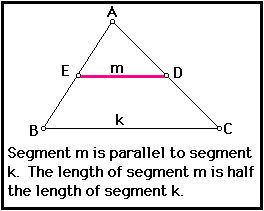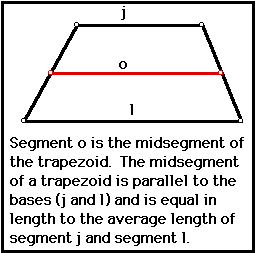# Midsegment Conjectures### Explanation:

A midsegment of a triangle is a segment connecting the midpoints of two sides of a triangle. This segment has two special properties. It is always parallel to the third side, and the length of the midsegment is half the length of the third side.

### The precise statement of the conjecture is:

Conjecture: (Triangle Midsegment Conjecture ): A midsegment of a triangle is parallel to the third side and is half the length of the third side.Extended Conjecture: (Trapezoid Midsegment Conjecture): The midsegment of a trapezoid is parallel to the bases and is equal in length to the average of the lengths of the two bases.Explanation: A midsegment of a trapezoid is a segment that connects the midpoints of the two non-parallel sides of a trapezoid. This segment has two special properties. It is parallel to the bases of the trapezoid. The length of the midsegment is the average of the lengths of the two bases. Remember that the bases of a trapezoid are parallel.

• Key Curriculum Press can provide demo versions of Geometer's Sketch Pad